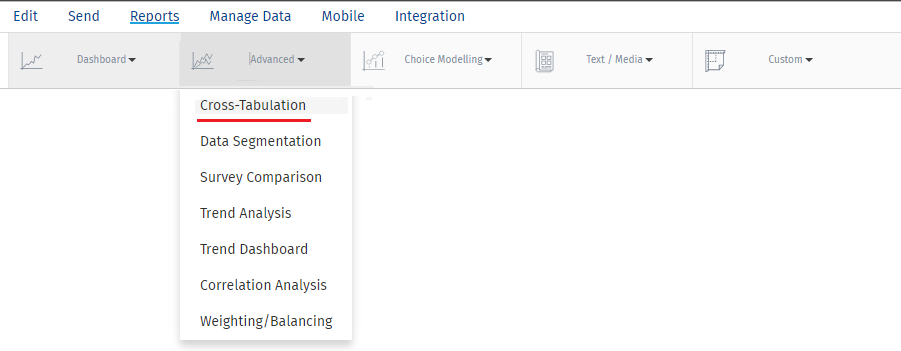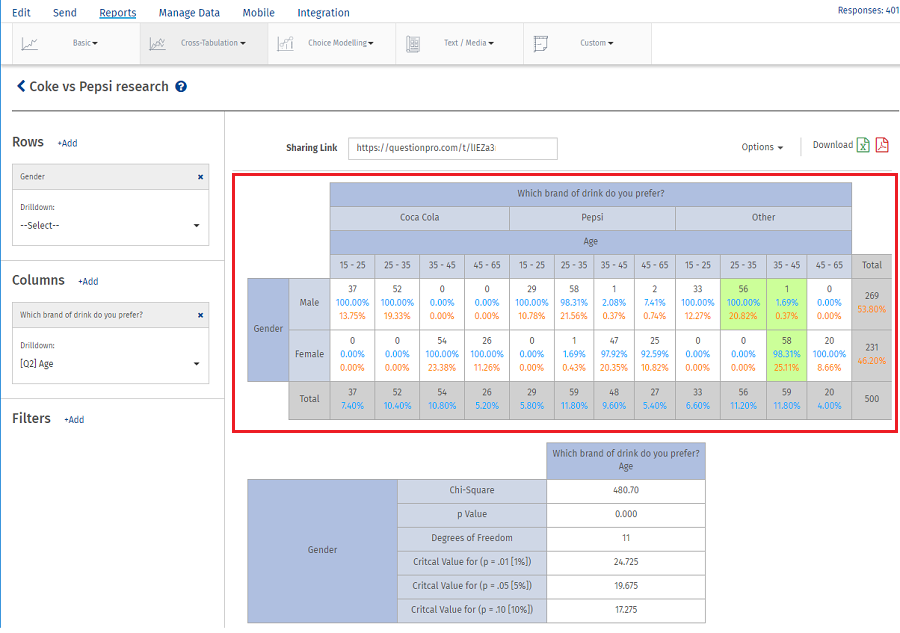# Cross Tabulation - Significant Cells

QuestionPro have opted cell comparison technique for highlighting the Most Significant cells. We consider the effect of other variables on the variable we study and see if there is any change in the percentage derived. Method opted for the calculation is column/cell comparison technique.

The cell highlighting option will only work if our Chi-Square Test confirms that there is an association between parent variables.

The highlighted cell would signify that the shown percentage is most significant when compared to other observation(It need not highlight the cell with the highest percentage).

This test will only confirm the cell is most statistically significant i.e. it is not affected by other variables and the percentage has not occurred as result of random chance variation.In above mentioned example we can read the data as:

1. Of total sample 269 of sample consist of male and 231 female categories.
2. Total sample could be again divided into 3 categories based on the drink they prefer which could be Coca Cola, Pepsi or Others.
3. These categories are then subdivided based on the age.
4. Highlighting of the significant cell will only appear if all these 3 variables (gender, drink and age) have an association between them which is confirmed by Chi-Square test.
5. For row Gender: Male, column Coca-Cola and Age: 15-25 the column percentage is shown to be 100% which is derived from (37/37).This calculation only takes into account Gender: Male, column Coca-Cola and Age: 15-25. It does not consider the impact of other columns which when considered may change this percentage. Hence we cannot say if the percentage derived is significant. To prove this we go for cell comparison wherein we take into account impact of all cells.

For Gender = Male, Drink = Coca-Cola and Age: 15-25 cell comparison calculation will be

(52+0+0+29+58+1+2+33+56+1+0)/(52+54+26+29+59+48+27+33+56+59+20)

Which comes to be 50.1%.

Now we calculate the p value between 100% and 50.1%.

Likewise we do the calculation for all the cells. we take mean of these p values. Any value below the mean is considered to be most significant variable and will be highlighted.

Since for Gender: Male, column Coca-Cola and Age: 15-25 there is no highlight of the cell this states percentage of the sample who prefer Coca Cola as the drink will vary when we consider the impact of other variables. Hence any promotional activity by the competitor like Pepsi or any other drink or even any other age category people can affect the buying decision of coke customers.